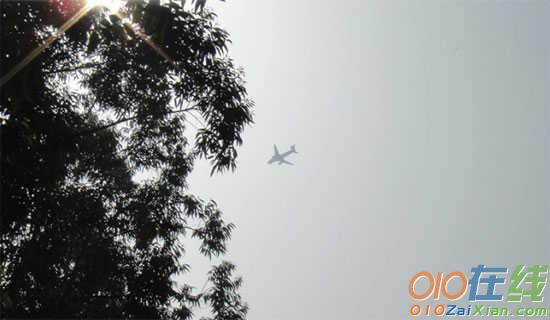## 9的乘法口诀练习题

一、口算。1×9=9×7=7×9=2×9=

9×3=4×9=6×9=5×9=

9×4=9×5=9×6=9×7=

二、把口诀填完整。

三九()()九得九()五十四

五九()()九三十六()六十三

四九()()九十八()八十一

三、()里最大能填几?

46>()×59×()<30

37>()×59×()<54

40>()×99×()<80

四、列式计算。

(1)4个9是多少?

(2)9的3倍是多少?

(3)7的9倍是多少?

(4)两个因数都是9，积是多少?